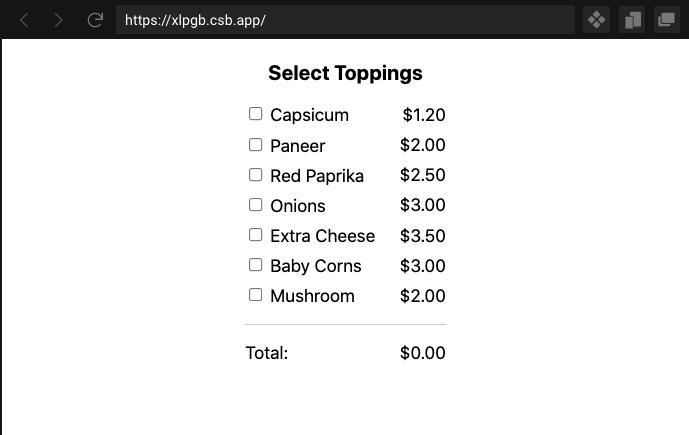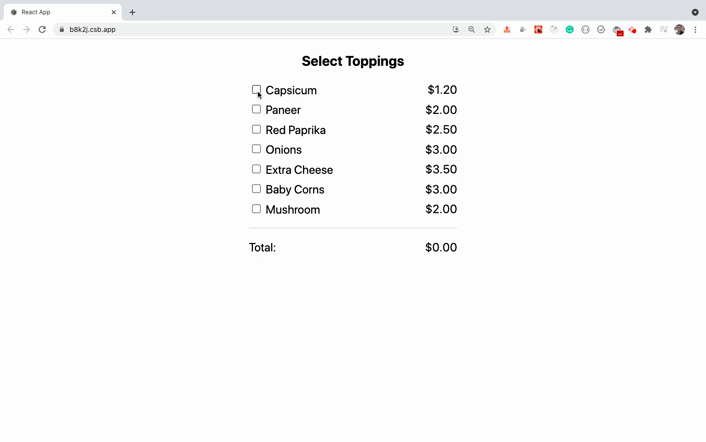# React 教程——如何使用多个复选框

• 如何在 React 中使用复选框作为受控输入
• 如何使用数组 map 和 reduce 方法进行复杂计算
• 如何创建预先填充了某些特定值的特定长度的数组

## 如何使用单个复选框

``````<div className="App">
<div className="topping">
<input type="checkbox" id="topping" name="topping" value="Paneer" />Paneer
</div>
</div>
````````````export default function App() {
const [isChecked, setIsChecked] = useState(false);

const handleOnChange = () => {
setIsChecked(!isChecked);
};

return (
<div className="App">
<div className="topping">
<input
type="checkbox"
id="topping"
name="topping"
value="Paneer"
checked={isChecked}
onChange={handleOnChange}
/>
Paneer
</div>
<div className="result">
Above checkbox is {isChecked ? "checked" : "un-checked"}.
</div>
</div>
);
}
``````

``````const [isChecked, setIsChecked] = useState(false);
``````

``````<input
...
checked={isChecked}
onChange={handleOnChange}
/>
``````

``````const handleOnChange = () => {
setIsChecked(!isChecked);
};
``````

## 如何处理多个复选框``````const [checkedState, setCheckedState] = useState(
new Array(toppings.length).fill(false)
);
``````

``````[false, false, false, false, false]
``````

``````import { useState } from "react";
import { toppings } from "./utils/toppings";
import "./styles.css";

const getFormattedPrice = (price) => `\$\${price.toFixed(2)}`;

export default function App() {
const [checkedState, setCheckedState] = useState(
new Array(toppings.length).fill(false)
);

const [total, setTotal] = useState(0);

const handleOnChange = (position) => {
const updatedCheckedState = checkedState.map((item, index) =>
index === position ? !item : item
);

setCheckedState(updatedCheckedState);

const totalPrice = updatedCheckedState.reduce(
(sum, currentState, index) => {
if (currentState === true) {
return sum + toppings[index].price;
}
return sum;
},
0
);

setTotal(totalPrice);
};

return (
<div className="App">
<h3>Select Toppings</h3>
<ul className="toppings-list">
{toppings.map(({ name, price }, index) => {
return (
<li key={index}>
<div className="toppings-list-item">
<div className="left-section">
<input
type="checkbox"
id={`custom-checkbox-\${index}`}
name={name}
value={name}
checked={checkedState[index]}
onChange={() => handleOnChange(index)}
/>
<label htmlFor={`custom-checkbox-\${index}`}>{name}</label>
</div>
<div className="right-section">{getFormattedPrice(price)}</div>
</div>
</li>
);
})}
<li>
<div className="toppings-list-item">
<div className="left-section">Total:</div>
<div className="right-section">{getFormattedPrice(total)}</div>
</div>
</li>
</ul>
</div>
);
}
``````

``````<input
type="checkbox"
id={`custom-checkbox-\${index}`}
name={name}
value={name}
checked={checkedState[index]}
onChange={() => handleOnChange(index)}
/>
``````

`handleOnChange` 程序方法如下所示：

``````const handleOnChange = (position) => {
const updatedCheckedState = checkedState.map((item, index) =>
index === position ? !item : item
);

setCheckedState(updatedCheckedState);

const totalPrice = updatedCheckedState.reduce(
(sum, currentState, index) => {
if (currentState === true) {
return sum + toppings[index].price;
}
return sum;
},
0
);

setTotal(totalPrice);
};
``````

``````const updatedCheckedState = checkedState.map((item, index) =>
index === position ? !item : item
);

// 上面的代码与下面的代码相同

const updatedCheckedState = checkedState.map((item, index) => {
if (index === position) {
return !item;
} else {
return item;
}
});
``````

``````<input
type="checkbox"
...
checked={checkedState[index]}
onChange={() => handleOnChange(index)}
/>
``````

``````const totalPrice = updatedCheckedState.reduce(
(sum, currentState, index) => {
if (currentState === true) {
return sum + toppings[index].price;
}
return sum;
},
0
);
``````• 如何在 React 中使用复选框作为受控输入
• 如何使用数组 map 和 reduce 方法进行复杂计算
• 如何创建预先填充了某些特定值的特定长度的数组

## 如何使用单个复选框

``````<div className="App">
<div className="topping">
<input type="checkbox" id="topping" name="topping" value="Paneer" />Paneer
</div>
</div>
````````````export default function App() {
const [isChecked, setIsChecked] = useState(false);

const handleOnChange = () => {
setIsChecked(!isChecked);
};

return (
<div className="App">
<div className="topping">
<input
type="checkbox"
id="topping"
name="topping"
value="Paneer"
checked={isChecked}
onChange={handleOnChange}
/>
Paneer
</div>
<div className="result">
Above checkbox is {isChecked ? "checked" : "un-checked"}.
</div>
</div>
);
}
``````

``````const [isChecked, setIsChecked] = useState(false);
``````

``````<input
...
checked={isChecked}
onChange={handleOnChange}
/>
``````

``````const handleOnChange = () => {
setIsChecked(!isChecked);
};
``````

## 如何处理多个复选框``````const [checkedState, setCheckedState] = useState(
new Array(toppings.length).fill(false)
);
``````

``````[false, false, false, false, false]
``````

``````import { useState } from "react";
import { toppings } from "./utils/toppings";
import "./styles.css";

const getFormattedPrice = (price) => `\$\${price.toFixed(2)}`;

export default function App() {
const [checkedState, setCheckedState] = useState(
new Array(toppings.length).fill(false)
);

const [total, setTotal] = useState(0);

const handleOnChange = (position) => {
const updatedCheckedState = checkedState.map((item, index) =>
index === position ? !item : item
);

setCheckedState(updatedCheckedState);

const totalPrice = updatedCheckedState.reduce(
(sum, currentState, index) => {
if (currentState === true) {
return sum + toppings[index].price;
}
return sum;
},
0
);

setTotal(totalPrice);
};

return (
<div className="App">
<h3>Select Toppings</h3>
<ul className="toppings-list">
{toppings.map(({ name, price }, index) => {
return (
<li key={index}>
<div className="toppings-list-item">
<div className="left-section">
<input
type="checkbox"
id={`custom-checkbox-\${index}`}
name={name}
value={name}
checked={checkedState[index]}
onChange={() => handleOnChange(index)}
/>
<label htmlFor={`custom-checkbox-\${index}`}>{name}</label>
</div>
<div className="right-section">{getFormattedPrice(price)}</div>
</div>
</li>
);
})}
<li>
<div className="toppings-list-item">
<div className="left-section">Total:</div>
<div className="right-section">{getFormattedPrice(total)}</div>
</div>
</li>
</ul>
</div>
);
}
``````

``````<input
type="checkbox"
id={`custom-checkbox-\${index}`}
name={name}
value={name}
checked={checkedState[index]}
onChange={() => handleOnChange(index)}
/>
``````

`handleOnChange` 程序方法如下所示：

``````const handleOnChange = (position) => {
const updatedCheckedState = checkedState.map((item, index) =>
index === position ? !item : item
);

setCheckedState(updatedCheckedState);

const totalPrice = updatedCheckedState.reduce(
(sum, currentState, index) => {
if (currentState === true) {
return sum + toppings[index].price;
}
return sum;
},
0
);

setTotal(totalPrice);
};
``````

``````const updatedCheckedState = checkedState.map((item, index) =>
index === position ? !item : item
);

// 上面的代码与下面的代码相同

const updatedCheckedState = checkedState.map((item, index) => {
if (index === position) {
return !item;
} else {
return item;
}
});
``````

``````<input
type="checkbox"
...
checked={checkedState[index]}
onChange={() => handleOnChange(index)}
/>
``````

``````const totalPrice = updatedCheckedState.reduce(
(sum, currentState, index) => {
if (currentState === true) {
return sum + toppings[index].price;
}
return sum;
},
0
);
``````• 如何在 React 中使用复选框作为受控输入
• 如何使用数组 map 和 reduce 方法进行复杂计算
• 如何创建预先填充了某些特定值的特定长度的数组

## 如何使用单个复选框

``````<div className="App">
<div className="topping">
<input type="checkbox" id="topping" name="topping" value="Paneer" />Paneer
</div>
</div>
````````````export default function App() {
const [isChecked, setIsChecked] = useState(false);

const handleOnChange = () => {
setIsChecked(!isChecked);
};

return (
<div className="App">
<div className="topping">
<input
type="checkbox"
id="topping"
name="topping"
value="Paneer"
checked={isChecked}
onChange={handleOnChange}
/>
Paneer
</div>
<div className="result">
Above checkbox is {isChecked ? "checked" : "un-checked"}.
</div>
</div>
);
}
``````

``````const [isChecked, setIsChecked] = useState(false);
``````

``````<input
...
checked={isChecked}
onChange={handleOnChange}
/>
``````

``````const handleOnChange = () => {
setIsChecked(!isChecked);
};
``````

## 如何处理多个复选框``````const [checkedState, setCheckedState] = useState(
new Array(toppings.length).fill(false)
);
``````

``````[false, false, false, false, false]
``````

``````import { useState } from "react";
import { toppings } from "./utils/toppings";
import "./styles.css";

const getFormattedPrice = (price) => `\$\${price.toFixed(2)}`;

export default function App() {
const [checkedState, setCheckedState] = useState(
new Array(toppings.length).fill(false)
);

const [total, setTotal] = useState(0);

const handleOnChange = (position) => {
const updatedCheckedState = checkedState.map((item, index) =>
index === position ? !item : item
);

setCheckedState(updatedCheckedState);

const totalPrice = updatedCheckedState.reduce(
(sum, currentState, index) => {
if (currentState === true) {
return sum + toppings[index].price;
}
return sum;
},
0
);

setTotal(totalPrice);
};

return (
<div className="App">
<h3>Select Toppings</h3>
<ul className="toppings-list">
{toppings.map(({ name, price }, index) => {
return (
<li key={index}>
<div className="toppings-list-item">
<div className="left-section">
<input
type="checkbox"
id={`custom-checkbox-\${index}`}
name={name}
value={name}
checked={checkedState[index]}
onChange={() => handleOnChange(index)}
/>
<label htmlFor={`custom-checkbox-\${index}`}>{name}</label>
</div>
<div className="right-section">{getFormattedPrice(price)}</div>
</div>
</li>
);
})}
<li>
<div className="toppings-list-item">
<div className="left-section">Total:</div>
<div className="right-section">{getFormattedPrice(total)}</div>
</div>
</li>
</ul>
</div>
);
}
``````

``````<input
type="checkbox"
id={`custom-checkbox-\${index}`}
name={name}
value={name}
checked={checkedState[index]}
onChange={() => handleOnChange(index)}
/>
``````

`handleOnChange` 程序方法如下所示：

``````const handleOnChange = (position) => {
const updatedCheckedState = checkedState.map((item, index) =>
index === position ? !item : item
);

setCheckedState(updatedCheckedState);

const totalPrice = updatedCheckedState.reduce(
(sum, currentState, index) => {
if (currentState === true) {
return sum + toppings[index].price;
}
return sum;
},
0
);

setTotal(totalPrice);
};
``````

``````const updatedCheckedState = checkedState.map((item, index) =>
index === position ? !item : item
);

// 上面的代码与下面的代码相同

const updatedCheckedState = checkedState.map((item, index) => {
if (index === position) {
return !item;
} else {
return item;
}
});
``````

``````<input
type="checkbox"
...
checked={checkedState[index]}
onChange={() => handleOnChange(index)}
/>
``````

``````const totalPrice = updatedCheckedState.reduce(
(sum, currentState, index) => {
if (currentState === true) {
return sum + toppings[index].price;
}
return sum;
},
0
);
``````